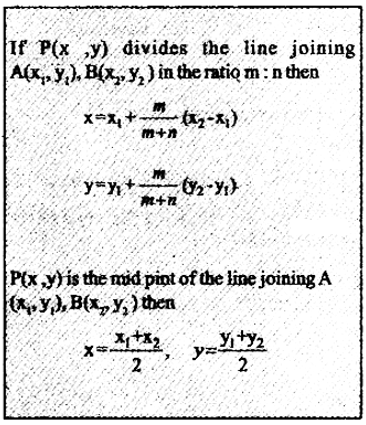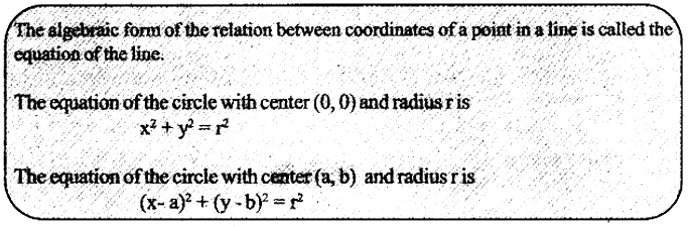# Kerala Syllabus 10th Standard Maths Solutions Chapter 9 Geometry and Algebra

## Kerala State Syllabus 10th Standard Maths Solutions Chapter 9 Geometry and Algebra

### Geometry and Algebra Textbook Questions & Answers

Textbook Page No. 213

Geometry and Algebra Class 10 Solutions Question 1.
What are the coordinates of the fourth vertex of the parallelogram shown on the right?Coordinate of the fourth vertex is = (6, 6)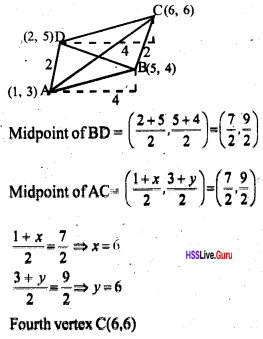Geometry and Algebra Class 10 Question 2.
The Figure shows a parallelogram with the coordinates of its vertices:
Prove that x1 + x3 = x2 + x4 and y1 + y3 = y2 + y4.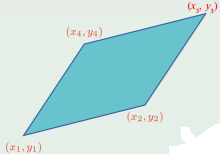Let P be the; point of intersection of diagonals of a parallelogram, which is the midpoint of AB and OC.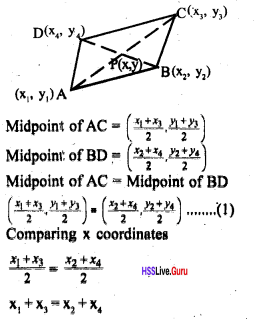From eqn (1)
Comparing y coordinates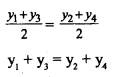Geometry And Algebra Class 10 Chapter 9 Kerala Syllabus Question 3.
A parallelogram is drawn with the lines joining (x1, y1) aid (x2, y2) to the origin as adjacent sides. What are the coordinates of the fourth vertex?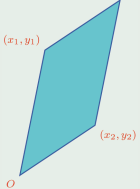Coordinates of the fourth vertex = (x1 + x2, y1 + y2)
Let P be the point of intersection of diago¬nals of a parallelogram, which is the midpoint of AB and OC.Sslc Maths Chapter 9 Kerala Syllabus Question 4.
Prove that in any parallelogram, the sum of the squares of all sides is equal to the sum of the squares of the diagonals.OA2 + OB2 = x12 + y12  + x22  + y22
OA = BC and OB = AC
OA2 + OB2 + OC2 + AC2 = 2(x12 + y12 + x22 + y22 )
AB2 = (x2 – x1 )2 + (y2 – y1 )2
OC2 = (x1 – x2)2 + (y2 – y3)2
OB2 + OC2 = 2(x12 + x22 + y12 + y22)
OA2 + OB2 + AC2 + BC2 = AB2 + OC2

Sslc Maths Geometry And Algebra Chapter 9 Kerala Syllabus Question 5.
In this picture, the midpoints of the sides of the large triangle are joined to make a small triangle inside. Calculate the coordinates of die vertices of the large triangle.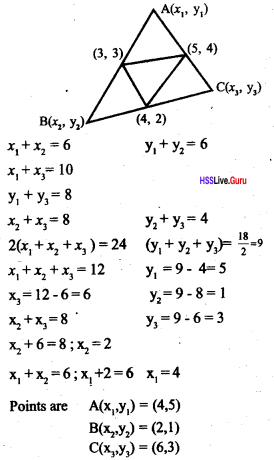textbook page no: 217

Sslc Geometry And Algebra Chapter 9 Kerala Syllabus Question 1.
A circle is drawn with the line joining (2, 3) and (6, 5) as diameter. What are the coordinates of the centre of the circle?
Midpoint of the line joining (2, 3) and (6, 5) isSslc Maths Chapter 9 Solutions Kerala Syllabus Question 2.
The coordinates of two opposite vertices of a parallelogram are (4, 5) and (1, 3). What are the coordinates of the point of intersection of its diagonals?
Midpoint of line joining the points (4, 5) and (1, 3) is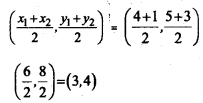The coordinates of the point of intersec¬tion of its diagonal is (3, 4).

Geometry And Algebra Class 10 Solutions Chapter 9 Kerala Syllabus Question 3.
The coordinates of the vertices of a quadrilateral, taken in order, are (2, 1), (5, 3), (8, 7), (4, 9).
i. Find the coordinates of the midpoints of all four sides.
ii. Prove that the quadrilateral got by joining these midpoints is a parallelogram.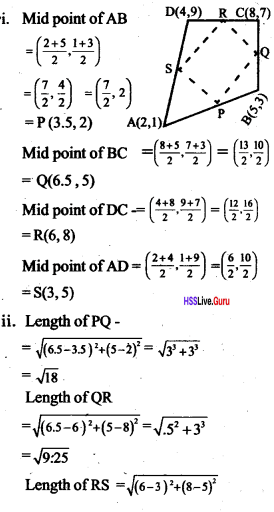Since the opposite sides of the quadrilateral PQRS are equal it is a parallelogram.

Geometry And Algebra Sslc Chapter 9 Kerala Syllabus Question 4.
In the figure, the midpoints of the large quadrilateral are joined to form the small quadrilateral within:
i. Find the coordinates of the fourth vertex of the smaller quadrilateral.
ii. Find the coordinates of the other three vertices of the larger quadrilateral.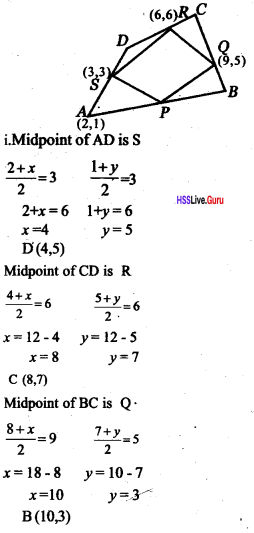Midpoint of AB is PCoordinate of the fourth vertex of smaller quadrilateral -(6, 2)
ii. Coordinates of other three vertices of the bigger quadrilateral (10, 3),(8, 7),(4, 5)

Geometry And Algebra Class 10 Kerala Syllabus Chapter 9 Kerala Syllabus Question 5.
The coordinates of the vertices of a triangle are (3, 5), (9, 13), (10, 6). Prove that this triangle is isosceles. Calculate its area.
IfA (3, 5), B (9, 13), C(10, 6)
are the vertices of the triangle ABC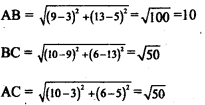As BC = AC the triangle is an isosceles triangleGeometry And Algebra Class 10 Pdf Chapter 9 Kerala Syllabus Question 6.
The centre of a circle is (1, 2) and a point on it is (3, 2). Find the coordinates of the other end of the diameter through this point
Midpoint of the diametertextbook Page no: 220

Geometry And Algebra Class 10 Questions And Answers Chapter 9 Kerala Syllabus  Question 1.
The coordinates of the points A and Bare (3, 2) and (8, 7). Find the coordinates of
i. the point P on AB with AP: PB = 2 : 3
ii. the point Q on AB with AQ: QB = 3: 2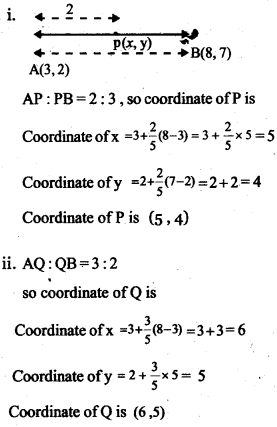Geometry And Algebra Class 10 Scert Chapter 9 Kerala Syllabus Question 2.
Find the coordinates of the points which divide the line joining (1, 6) and (5, 2) into three equal parts.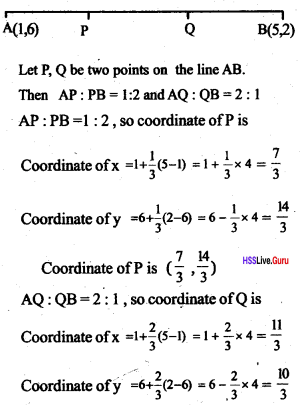Coordinate of Q is $$\left(\frac{11}{3}, \frac{10}{3}\right)$$

Algebra Solutions Question 3. The coordinates of the vertices of a triangle are (-1, 5), (3, 7), (1, 1). Find the coordinates of its centroid.
Centroid of the triangle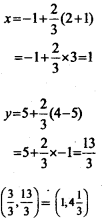Question 4.
Calculate the coordinates of the point Pin the picture:Bisector of an angle divides the opposite side in the ratio of the sides containing the angle.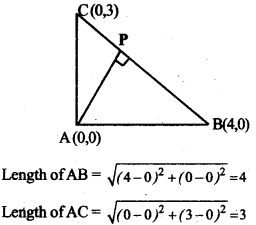So, the coordinates of the point P which divides the line joining (4, 0) and (0, 3) in the ratio 4 : 3.
BP : PC = 4 : 3, so coordinate of P is Coordinate of x $$=4+\frac{4}{7}(0-4)=4-\frac{16}{7}=\frac{12}{7}$$
Coordinate of y $$=0+\frac{4}{7}(3-0)=0+\frac{12}{7}=\frac{12}{7}$$
$$\text { Coordinate of } P \text { is }\left(\frac{12}{7}, \frac{12}{7}\right)$$

Textbook Page No. 227

Question 1.
Prove that the points (1, 3), (2, 5), (3, 7) are on the same line.
Coordinates of slope of a line, which joins the points (1, 3) and (2, 5) = $$\frac{5-3}{2-1}=2$$
Slope of a line, which joins the points (2, 5) and (3, 7) $$=\frac{7-5}{3-2}=2$$
both have same slope, so they make equal angles with the x-axis.
∴ They all lie on the same line.

Algebra 10th Standard Question 2. Find the coordinates of two more points on the line joining (-1, 4) and (1, 2).
Change in x is 2 and y is -2
When change in x be 1 then change in y = $$\frac{-2}{2}=-1$$
Let change in x be 1 then change in y will be 3.
Coordinate of y = 4 + 3 x – 1 = 1
Point (2, 1)
Let change in x be 3 then change in ywillbe 4.
∴ Coordinate of y = 4 + 4 x – 1 = 0
∴ Point (3, 0)
Two points are (2, 1), (3, 0)

Question 3.
x1, x2, x3, ……… and y1, y2, y3, …….. are arithmetic sequences. Prove that all the points with coordinates in the sequence (x1, y1), (x2, y2), (x3, y3) ……… of number pairs are on the same line.
If the line which contains the points (x1, y1), (x3, y3) also contains (x, y), then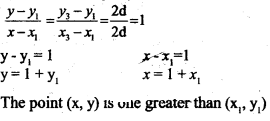Therefore, (x1, y1), (x2, y2) …………. will be in the same line

Question 4.
Prove that If the points (x1, y1), (x2, y2), (x3, y3) are on a single line, then the points (3x1 + 2y1, 3x1 – 1y1), (3x2 + 2y2, 3x2 – 2y2), (3x3 + 2y3, 3x3 – 2y3) are also on a single line. Would this be true if we take some other numbers instead of 3 and 2?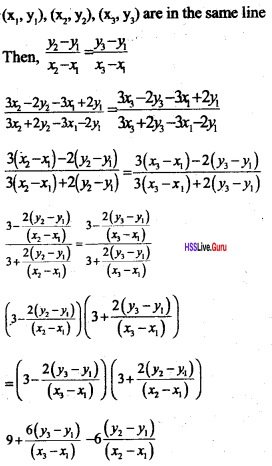Yes it is possible.

Textbook Page no: 231

Question 1.
Find the equation of the line joining (1, 2) and (2, 4). For points on this line with consecutive natural numbers 3, 4, 5, ……… as the x coordinates, what is the sequence of y coordinates?
Equation of line joining the points (1, 2), (2, 4)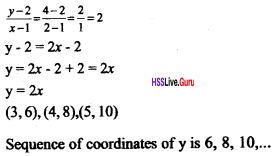Question 2.
Find the equation of the line joining (–1, 3) and (2, 5). Prove that if the point (x, y) is on this line, so is the point (x+3, y+2).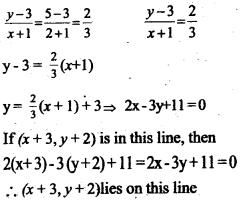Question 3.
Prove that whatever number we take as, the point (x, 2x + 3) is a point on the line joining (–1, 1) and (2, 7).= $$\frac{2 x+3-7}{x-2}=\frac{2 x-4}{x-2}=2$$
The slopes are same hence die point (x, 2x+3) is passing through the points (–1, 1), (2, 7)

Question 4.
The x coordinate of a point on the slanted (blue) line is 3:
i. What it its y coordinates?
ii. What is the slope of the line?
iii. Write the equation of the line.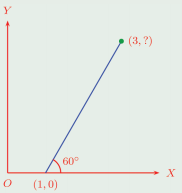As we get a right triangle on joining die three points’, with angles 30 : 60: 90 and ratio of their sides 1 : √3: 2Question 5.
In the picture here, ABCD is a square. Prove that for any point on the diagonal BD, the sum of the x and coordinates is zero.B(2, -2), D(-2, 2) are the vertices of the diagonal BD: Sum of x,y coordinates of B = 2 + –2 = 0
Sum of x,y coordinates of D = –2 + 2 = 0
If (x, y)is a point on BD, thenQuestion 6.
Prove that for any point on the line intersecting the axes in the picture, the sum of the x and y coordinates is 3.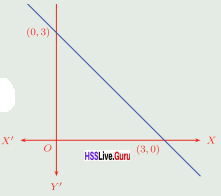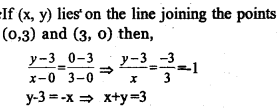∴ the sum of the and y coordinates of the point is 3.

Question 7.
Find the equation of the circle with center at the origin and radius 5. Write the coordinates of eight points on this circle.
Equation of the circle
= (x – 0)2 + (y – 0)2 = 52
= x2 + y2 = 25
x2 = 25 – y2
x = $$=\sqrt{25-y^{2}}$$
y = 0 x = 5 (5, 0), (–5, 0)
y = 5 x = 0 (0, 5), (0, –5)
y = 3 x = 4 (4, 3), (4, 3)
y = 4 x = 3 (3, 4), (3, –4)
Coordinates of points on the circle
(5, 0), (–5, 0), (0, 5), (0, -5), (4, 3), (4, 3), (3, 4), (3, 4).

Question 8.
Prove that if (x, y) be a point on the circle with the line joining (0, 1) and (2, 3) as diameter, then x2 + y2 – 2x – 4y + 3 = 0. Find the coordinates of the points where this circle cuts they axis.
As (0, 1), (2, 3) are the points joining the diameter of the circle, the center will beLet (x, 0) be the coordinate which intersect
the x axis then, x2 – 2x +3=0
$$x=\frac{2 \pm \sqrt{4-12}}{2}=\frac{2 \pm \sqrt{-8}}{2}$$
This circle can not be intersect the x axis. Let (0, y) be the coordinate which intersect the y axis then, y2 – 4y + 3 = 0,
(y – 3)(y – 1) = 0 Coordinates are (0, 1) and (0, 3)

Question 9.
What is the equation of the circle shown here?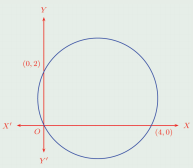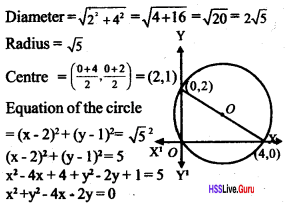### Geometry and Algebra Orukkam Questions & Answers

Worksheet 1

Question 1.
If A(2, –1), B(3, 4), C(–2, 3) are the vertices of a triangle find the fourth vertex.
Difference between x coordinates of A and B = 3 – 2 = 1, Coordinate of y = 4 + 1 = 5
x coordinates of D = –2 – 1 = -3
y coordinates of D = 3 – 5 = –2
Coordinates of D = (–3, –2)

Question 2.
In triangle ABC (4, 2) is the midpoint of AB. The midpoint of BC is (5, 4), the midpoint of AC is (3, 3). Find the vertices of the triangle.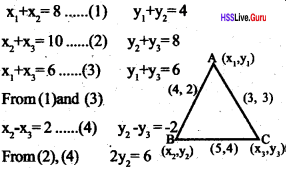2x2 = 12
∴ x2 = 6
∴ y2 = 3
From(1) y1 = 4 – 3 = 1
x = 8 – 6 = 2, y3 = 6 – 1 = 5
From(3) x3 = 6 – 2 = 4 ,
Coordinates of A, B and C A(2, 1), B(6, 3), C(4, 5)

Question 3.
If A(2, –2), B(14, 10), C(11, 13) are the three vertices of a parallelogram ABC D write the coordinates of the fourth vertex
A(2, –2), B(14, 10), C(11, 13).
Difference of x coordinates of A and B = 14 – 2 = 12.
x coordinate of D = 11 – 12 = 1.
Difference of y coordinates of A and B= 10 – (–2) = 12
y coordinates of D =13 – 12 = 1
Coordinates of D = (–1, 1)

Worksheet 2

Question 4.
Find the coordinates of the point which divides the line joining(4, –3), (9, 7) in the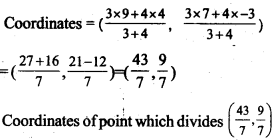Question 5.
Find the coordinates of the midpoint of the line joining the points(1, –2), (–3, 4)
Coordinates of midpoint =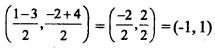Question 6.
The points A(6, 1), B(8, 2), C(9, 4), D(p, 3) are the vertices of a parallelogram. Find the value of p using the concept that the diagonals of a parallelogram bisect each other.The x coordinate of O using the concept that the diagonals of a parallelogram bisect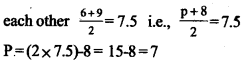Question 7.
One end of the diameter of a circle is (1, 4). The center of the circle is (3, –4). Find the coordinates of other end
One end of the diameter = (x1, y1) = (1, 4)
Center of the circle = (x, y) =(3, -4)
If other end of the diameter = (x2, y2)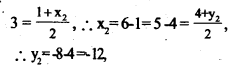Other end of the diameter (5 – 12)

Question 8.
Find the coordinates of the points P and Q which trisect the line joining (2, –3), and (4, –1) P,Q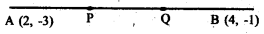P can divide the line which joins the points (2, –3),(4, –1)in the ratio of 1 : 2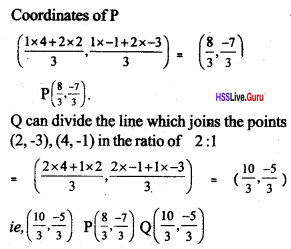Question 9.
Prove thatA(6, 4), B(5, –2), C(7, –2) are the vertices of an isosceles triangle. If D is the midpoint of the side BC ,find the coordinates of D. Calculate the length of this median. Also, find the coordinates of centroid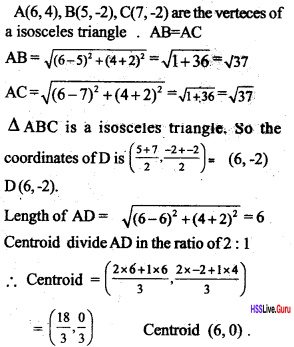Worksheet 3

Question 10.
A-line makes an angle 45° with x-axis.Find the slope of the line
Slope of the line = tan 45° = 1Question 11.
The points on a line are (–1, 1), (3, 1), (5, 1)What is the angle made by this line with x-axis? What is the slope of this line
This line is parallel to the x-axis so the slope is 0.

Question 12.
Find the slope of the line passing through (1, –3) (3, –5).
Slope = $$\frac{-5+3}{3-1}=\frac{-2}{2}=1$$

Question 13.
A line passing through a point at a distance 4 from the right of origin on x-axis. If (3, –5) is a point on this line, find the equation of the line.
Points are (4, 0) (3, –5)
Slope = $$\frac { -5 }{ -1 }$$ = 5

Question 14.
If a line cut x-axis at (5, 0) and y-axis at (0, –3) Find the slope of the line given below.
Slope of the line = $$\frac{-3-0}{0-5}=\frac{-3}{-5}=\frac{3}{5}$$

Question 15.
Find the slope of the line given below.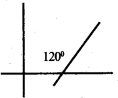Slope of the line = tan 60° = √3

Worksheet 4

Question 16.
Prove that the points (1, 3) (2, 5) (3, 7) are on a lineThree points are lies on the same line.

Question 17.
The numbers in the sequence 2, 5, 8,11 and the numbers in the sequence 7, 11, 15, 19 …. are joined pairwise as given below(2, 7), (5, 11), (8, 15) Prove that these are on a line.
Distance between (2, 7) and (5, 11) = $$=\sqrt{9+16}=5$$
Distance between (5, 11) and (8, 15) = $$=\sqrt{9+16}=5$$
Hence the distances are same, so the points are lie on the same line.

Question 18.
Find the slope of the line passing through (–2, 3) (5, 7) Write the slope of the fine parallel to it.
Slope of the line which passing through the points (–2, 3) and (5, 7) = $$\frac{7-3}{5+2}=\frac{4}{7}$$
The line which joins the points (–2, 2) and (5, 6) is parallel to the first line.
Slope of the line which passes through the points (–2, 2) and (5, 6) = $$=\frac{6-2}{5+2}=\frac{4}{7}$$
Slope of the line which passes through the points (–7, 4) and (0, 8) = $$=\frac{8-4}{0+7}=\frac{4}{7}$$
The lines are parallel because their slopes are same.

Worksheet 6

Question 19.
Find the equation of the line passing through (5, 1), (1, –1), (11, 4)
Equation of the line which passes through the points (5, 1), and (1, –1)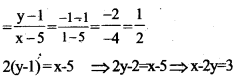Equation is x – 2y – 3 = 0
Let find (11, 4) be on the equation 11 – 2 x 4 – 3 = 11 – 8 – 3 = 0
Equation is x – 2y – 3 = 0

Question 20.
(3, 0) is a point on the line joining the points (3x2, 6x), (3y2, 6y) then prove that xy = –1
Slope of the line which passing through the points (3x2, 6x) and (3y2, 6y)That is equal to the slope of the line which joins the points (3, 0) and (3x2, 6x).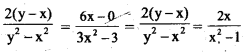Worksheet 7

Question 21.
If a line is passing through (1, 1). This point divides the segment between axes in the ratio 3 : 4. Find the equation of this line.
Let consider the line 4x + 3y = 7, which cuts x axis at (a, 0) and y axis at (0, b)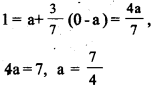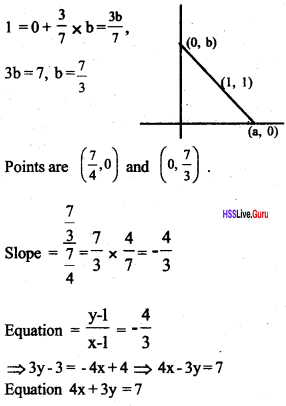### Geometry and Algebra SCERT Questions & Answers

Question 22.
In the parallelogram ABCD A(2, 3); B(7, 3); and D(4, 7). Find the coordinates of C. [Score: 4, Time: 6 minutes]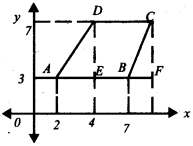In the figure ΔAED, ΔBFC
AD = BC. DE = CF (1)
AE = BF = 2 unit (1)
∴ x coordinate of C
= 7 + BF = 7 + AE = 7 + 2 = 9 (1)
y coordinate of C = 3 + FC = 3 + ED = 3 + 4 = 7 (1)
C has the coordinates (9, 7)

Question 23.
In parallelogram ABCD A(2, 3); B(6, 5); and D(4, 7). Find the coordinates of C [Score: 4, Time: 6 minutes]Draw AE, DF parallel to x-axis. (1)
Draw BE, CF parallel to y-axis.
In right triangles Δ ABE, Δ DCF (1)
AB=DC, ∠BAE = ∠CDF
∠ABE = ∠DCF
∴ AE = DF= 6 – 2 = 4
BE = CF =5 – 3 = 2
x coordinate of C = 4 + DF = 4 + 4 = 8
y coordinate of C = 7 + CF = 7 + 2 = 9
Coordinates ofC (8, 9) (1)

Question 24.
In parallelogram ABCD A(x1, y1),B(x3, y2), D(x2, y3) Find the coordinates of C. [Score: 4, Time: 6 minutes]Draw AE, DF parallel to x-axis
Draw BE, CF parallel to y-axis
In right triangles ΔAEB, ΔDFE
AB=DC (1)
∠BAE = ∠CDF ∠ABE = ∠DCF
∴ AE = DF = x2 – x1 (1)
BE = CF = y2 – y1 (1)
x coordinate of C =
x3 + DF = x3 + x2 -x,
y coordinate of C = y3 + CF = y3 + y2 – y1
∴ C has the coordinates =(x2 + x3 – x1, y2 + y3 – y3) (1)

Question 25.
Find the coordinates of the fourth vertex of the parallelogram shown here. [Score: 2, Time: 6 minutes]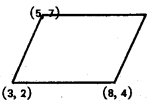If A(x1, y1); B(x3, y2); D(x2, y3) are vertices of a parallelogram ABCD, then C has the coordinates (x3 + x2 – x1, y3 + y2 – y1) (1)
x coordinate of fourth vertex = 8 + 5 – 3 = 10
y coordinate of fourth vertex = 7 + 4 – 2 = 9
Coordinate of fourth vertex (10, 9) (1)

Question 26.
In the parallelogram PQRS, P(-3, 2) Q(2, 7), S(1, 9) are three vertices. Find the length of the diagonal PR. [Score: 4, Time: 6 minutes]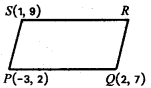x coordinate of R = 2 + 1 – (–3) = 6 (1)
y coordinate of R = 9 + 7 – 2 = 14 (1)
(6, 14) is the coordinate of R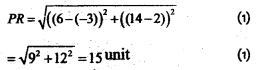Question 27.
A ABC A(6, 8); B(3, 4); C(–2, 2) are its vertices. The bisector of ∠A cuts BC at D.
a Find BD: CD.
b. Find the coordinates of D. [Score: 4, Time: 8 minutes]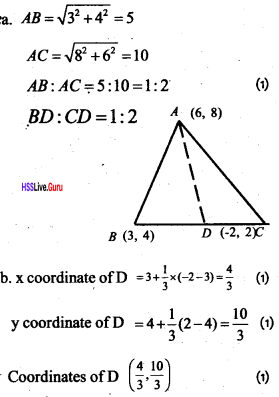Question 28.
A circle is drawn with AB as diameter whose endpoints are A(3, 1) and 5(9, 10)
a. Find the coordinates of the center of the circle.
b. If another circle with diameter one-third of the above circle is drawn with the same center, what are the points that the circle cuts AB? [Score: 5, Time: 8 minute]
a. Coordinates of the center OA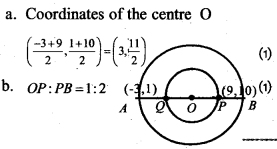### Geometry and Algebra Exam Oriented Questions and Answers

Short Answer Type Questions (Score 2)

Question 29.
Find the midpoint of the line segment joining the points(3, 0), (–1, 4).
Mid point of the line segment joining the line segment joining the points (3, 0), (–1, 4).
$$=\left(\frac{3-1}{2}, \frac{0+4}{2}\right)=(1,2)$$

Question 30.
Find the equation of the line joining (1, 3), (2, 7).
If (x, y) be any point on this line, then
$$\frac{(y-4)}{(x-1)}=2$$
y – 4 = 2(x – 1)
y – 4 = 2x – 2
2x + y + 2 = 0

Question 31.
What is the distance between the origin and the point (–2, -3)? What is the relation between this distance and the distance between the origin and the point (2, 3)?
The distance to the points (–2,–3) is $$\sqrt{(-2)^{2}+(-3)^{2}}=\sqrt{13}$$
The distance to the points (2, 3) is $$\sqrt{2^{2}+3^{2}}=\sqrt{13}$$
Both the distances are equal.

Question 32.
Mark the points A(2, 3), 6(6, 6) by drawing the axes. Draw a rectangle with AB as di-agonal and sides are parallel to the axes. Find the coordinates of the other vertices of the rectangle. Find the length and breadth of the rectangle. Find the length of the diagonal AB.
P(6, 3) Q(2, 6)
length = 4, breadth = 3
AB = $$\sqrt{4^{2}+3^{2}}-\sqrt{25} \div 5$$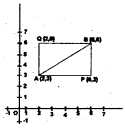Question 33.
What is the equation of the circle? Centre (2, 1), radius √5.
Equation of the circle
(x – 2)2 + (x – 1)2 = 5
x2 – 4x + 4 + y2 – 2y + 1 = 5
x2 + y2 – 4x – 2y = 0

Question 34.
If (7, 3), (6, 1), (8, 2) and (p, 4) are the vertices of a parallelogram taken in order, then find the value of p.The midpoint of the diagonal AC and the diagonal BD coincide.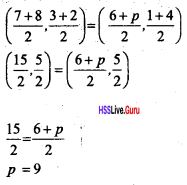Question 35.
Find the equation of the line joining (–1, 3), (2, 5).Short Answer Type Questions (Score 3)

Question 36.
A is a point on the Y axis, equidistant from (3, 5) and (2, 6). Draw a rough sketch. If the Y co-ordinate of Ais P. What are the coordinates of A, in terms of P? Calculate the Value of P and hence the coordinates of A.
The coordinate of A is (0, P). Since A is a point on the y-axis.P2 – 12P + 40 = P2 – 10P +34
P2 – P2 – 12P + 10P = 34 – 40 – 2P = -6
P=3
∴ Coordinates of A are (0, 3)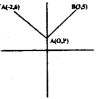Question 37.
The points A(0, 0), B(10, 0), C(5, 5√3 ) are the vertices of the triangle ABC. Find AB, BC, AC and prove that the triangle is equilateral.
A(0, 0), B(10, 0), C (5, 5√3)
AB = 10 unit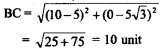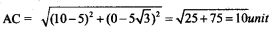AB = BC = AC
∴ ΔABC is equilateral

Question 38.
Prove that the points (2, 3), (7, 5), (9, 8), (4, 6) are the corners of a parallelogram.Since opposite sides are parallel, ABCD is a parallelogram.

Question 39.
a. What is the point at which the line 2x + 4y – 1 = 0 cuts the x-axis?
a. If the line 2x + 4y – 1 = o cut the x -axis at (x, 0), then 2x – 1 = 0
x = 1/2
The line intersect x axis is ( 1/2, 0 )
If the line 2x + 4y – 1 = 0 cut the y-axis at (0, y), then
4y – 1 = 0
y = 1/4
The line intersect y axis is (0, 1/4).

Long Answer Type Questions (Score 4)

Question 40.
Find the slope of the line joining (2, 3) and (3, –1). Check whether this line passes through the point (5, 6). What about the point (5, -9)?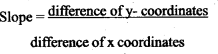∴ Slope of the line joining (2, 3) and (3, –1)
$$=\frac{3-1}{2-3}=\frac{3+1}{-1}=-4$$
Slope of the line is –4
For point (5, –6)
Slope = $$\frac{6-3}{5-2}=\frac{3}{3}=1$$
Since slope = 4,
this line does not pass through(5,6)
For point (5, –9) Slope = $$\frac{-9-3}{5-2}=\frac{-12}{3}=-4$$
The line (5, –9) pass through the point.

Question 41.
Write the coordinates of each points on the lines 2x – y + 1 = 0 and 3x – 2y + 3 = 0. Find the co-ordinates of the point of intersection of the lines.Question 42.
a. Find the slope of the line joining the points (5, 3) and (4, 1).
b. Which are the points where this line meets x-axis and y-axis?
c. Write the coordinates of two other points on the line.
a. Let (5, 3) = (x1, y1) and (4, 1) = (x2, y2),
= slop = $$\frac{y_{2}-y_{1}}{x_{2}-x_{1}}=\frac{1-3}{4-5}=\frac{-2}{-1}=\frac{2}{1}=2$$
b. If the line meets x axis at the point (x, 0), then slope = $$\frac{a-3}{x-5}=2 \Rightarrow 2(x-5)=-3$$
2x – 10 = –3,
2x = –3 + 10 = 7,
x = 7/2 = 3.5,
point is = (3.5, 0)
y axis $$\frac{y-3}{0-5}=2 \text { point }(0,-7)$$

c. slope is (2/1) means when x coordinate increase/decreases by 1, y co-ordinate increases decreases by 2. Another point on the line is (5 + 1), (3 + 2) = (6, 5)
A third point = (6 + 1) (5 + 2) = (7, 7)

Long Answer Type Questions (Score 5)

Question 43.
In the figure, the center of the circle is the origin and its radius is 1 unit. The points A,B are on the circle with ∠AOP = 30°, ∠AOB = 15°.
a. Find the coordinates of A and B.
b. Find the relation between the slope of the lines OA, OB and the tan measures of angles they make with the x-axis.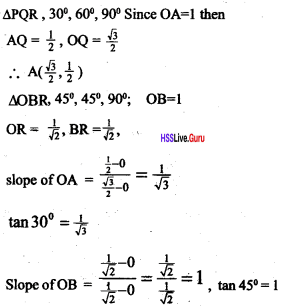Slopes of the lines and die tangent values-of the angles made by the lines with the axis are equal.

Question 44.
A circle is drawn with origin as the center and radius 10 units. Show that the point (–8, –6) is a point on the circle. Find out whether the point (9, –1) lies within the circle or outside the circle. Why?
The distance between the centre and the point (-8,-6) is = $$=\sqrt{(-8)^{2}+(-6)^{2}}=\sqrt{100}=10$$
It is equal to the radius. So the point (-8, -6) is a point on the circle.
The distance between the centre, and the point (9,-1) is = $$\sqrt{9^{2}+(-1)^{2}}=\sqrt{81+1}=\sqrt{82}$$
√82 is smaller than 10.
So the point (9, –1) is a point inside the circle.

### Geometry and Algebra Memory Map

Distance between (x1,y1) and (x3, y2) is $$\sqrt{\left(x_{2}-x_{1}\right)^{2}+\left(y_{2}-y_{1}\right)^{2}}$$
Distance of (x, y) from (0, 0) is $$\sqrt{x^{2}+y^{2}}$$